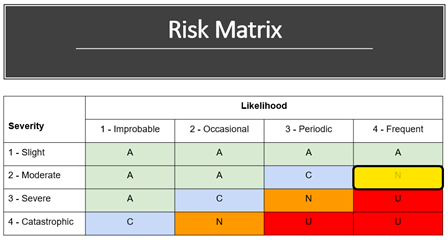### Risk Ranking Matrix (Part 6)

In order to objectively rate the risk of each scenario that is considered, most PHAs utilize a risk ranking matrix. The concept of risk is the product of the severity of the event occurring and the likelihood of its occurrence. The matrix that we typically use at Resource Compliance has severity on the vertical axis and likelihood on the horizontal axis. In our matrix, a ranking of ‘1’ is the least severe or least likely and a ranking of ‘4’ is the most severe or most likely to occur.To illustrate how the matrix works, consider a scenario that has a severity of ‘2’ and a likelihood of ‘4’. As displayed below, the corresponding risk is ‘N’. The matrix that we often use, has the following risk definitions:

• A: Acceptable – No risk control measures are needed
• C: Acceptable with Control – Risk control measures are in place
• N: Not Desirable – Risk control measures to be introduced within a specified time period
• U: Unacceptable – Risk control measures to be completed at the earliest possible opportunityEach team member should be familiar with the risk ranking matrix prior to beginning the PHA.

This is Part 6 of a series on PHAs. You can access previous blogs in this series below: